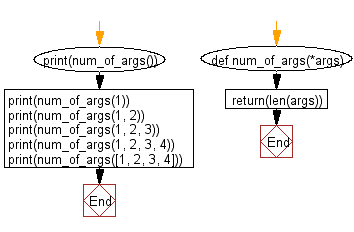﻿ Python: Count the number of arguments in a given function - w3resource

# Python: Count the number of arguments in a given function

## Python Basic - 1: Exercise-91 with Solution

Write a Python program to count the number of arguments in a given function.

Sample Solution:

Python Code:

``````def num_of_args(*args):
return(len(args))
print(num_of_args())
print(num_of_args(1))
print(num_of_args(1, 2))
print(num_of_args(1, 2, 3))
print(num_of_args(1, 2, 3, 4))
print(num_of_args([1, 2, 3, 4]))
``````

Sample Output:

```0
1
2
3
4
1
```

Pictorial Presentation:Flowchart:Python Code Editor:

Have another way to solve this solution? Contribute your code (and comments) through Disqus.

What is the difficulty level of this exercise?

Test your Programming skills with w3resource's quiz.

﻿